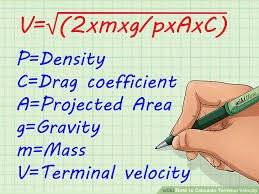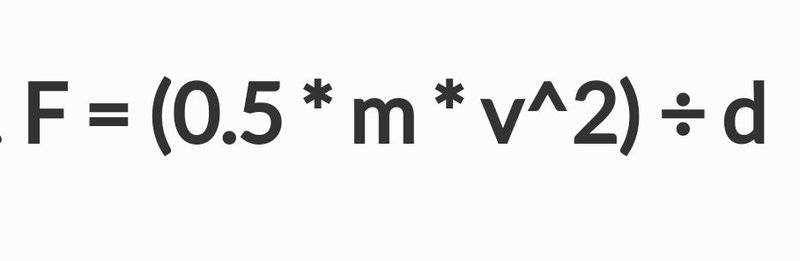# Force of a free-falling body

Tankoyu
I am trying to find out the amount of force that a free-falling body will have, as it hits the ground from a distance of 2000 km from Earth's surface.(Considering air resistance)

What I know about the body:

Area of the body=20199.04 mtr²
Density of body=985 Kg/m³
Thickness of body= 75 mtr.

After scaling the area 10 times,(since the actual body is 10 times larger)

Area of the scaled body=2019904 mtr²

Volume of body: area*thickness

Therefore, volume=2019904*75=151492800 mtr³

Mass= volume*density
Mass= 151492800*985
= 149220408000 kg

Terminal velocity of body:Density of air=1.225 kg/m³
Drag coefficient of the body, C =1.98

Using the formula for terminal velocity,

Vt=773 mtr/sec (~ 2.25 Mach)

Force of impact,'V' is taken as the value of terminal velocity (Vt)

Using the above formula, I have found the force,

F= 1,463,766,251,524.9 kgf

Or = 14,354,643,310,487 N

Can anyone help me validate this value??

Have I used the proper procedure to achieve the force value?

#### Attachments

Last edited:

Staff Emeritus
Homework Helper
Gold Member
First of all, you are using way too many significant digits. Your final result contains 14(!) significant digits, which would indicate that you know all input values with a relative accuracy of the size of a proton compared to a meter.

Second, it is not clear what you consider ”amount of force” to be, or that you understand what should go into your formula. Can you describe in words what all parts of the formula describe? If not, then you are likely just stabbing in the dark, which is never a good approach to have. It is better to understand what goes into the expressions and what they are saying.

•sophiecentaur
Tankoyu
First of all, you are using way too many significant digits. Your final result contains 14(!) significant digits, which would indicate that you know all input values with a relative accuracy of the size of a proton compared to a meter.

Second, it is not clear what you consider ”amount of force” to be, or that you understand what should go into your formula. Can you describe in words what all parts of the formula describe? If not, then you are likely just stabbing in the dark, which is never a good approach to have. It is better to understand what goes into the expressions and what they are saying.
By amount of force, I mean: how much of force does the free falling body carry before it strikes the surface of Earth.

And all those formulae are taken from internet so I'm not sure if they are applied in the right context[emoji28]

And what do you suggest I do about the value, assuming it is correct?

Staff Emeritus
Homework Helper
Gold Member
By amount of force, I mean: how much of force does the free falling body carry before it strikes the surface of Earth.
It is still not clear what you mean by "much of force does the free body carry". What force on what object are you trying to compute? A force is a transfer of momentum per time from one system to another.

And all those formulae are taken from internet so I'm not sure if they are applied in the right context[emoji28]
I strongly suggest against just taking formulae without understanding the appropriate context. It will tell you nothing. In order to get something out of the exercise you need to understand what you are doing.

And what do you suggest I do about the value, assuming it is correct?
Regardless of whether it is correct or not, which is impossible to say without knowing what you have done or what you want to compute, you should always give your final results with an appropriate number of significant digits. In order to do this, round the result to the closest number that has the correct number of significant digits.

•sophiecentaur
Mentor
To add to @Orodruin's answer: bodies do not have forces, they have momentum.

•sophiecentaur
Homework Helper
Gold Member
Objects don't "carry" a force. They have forces applied to them or they apply force on other objects.

What they do "carry" is kinetic energy.

You can calculate an average force that they apply on the ground if you know the kinetic energy and "stopping distance". However this method isn't really applicable to something impacting the ground from space because it has more in common with an explosion than an impact.

Tankoyu
It is still not clear what you mean by "much of force does the free body carry". What force on what object are you trying to compute? A force is a transfer of momentum per time from one system to another.

I have not been using the correct terms, I regret that. Thank you for answering me,

What I want to compute is, as the free falling body hits the surface of Earth & comes to a rest- how much of the momentum does the object impart to earth? How can I find out the amount of energy transferred in terms of Force?

how much of the momentum does the object impart to earth? How can I find out the amount of energy transferred in terms of Force?
Momentum, energy and force are different quantities. Which are you interested in?

Tankoyu
Momentum, energy and force are different quantities. Which are you interested in?
Force

Staff Emeritus
Homework Helper
Gold Member
Ok, so which force? To specify a force you need: A system that the force acts upon, a system that the force acts from, additional assumptions about when you want to compute that force.

Tankoyu
Ok, so which force? To specify a force you need: A system that the force acts upon, a system that the force acts from, additional assumptions about when you want to compute that force.
I'm not sure, I understand what you asked...

Gold Member
Force of impact,This formula is 'not incorrect' (note the double negative) but you need to think what it actually refers to. The d in the equation refers to the distance, under a constant force, over which the work done is equal to the Kinetic Energy of the object on impact and the object is brought to rest. So, unless you know the distance traveled by the object as it is brought to a halt in the concrete / soft mud / water / crash mat and unless you can be sure the force is the same throughout the impact, the value F can't be known.
This is why you have already been told in this thread that the most meaningful quantities are Momentum or Kinetic Energy. They are properties of your falling object and don't depend on what it lands on. Momentum and KE are alternative ways of looking at 'that thing' a moving object posses.

#### Attachments

•CWatters and Tankoyu
Staff Emeritus
Homework Helper
Gold Member
I'm not sure, I understand what you asked...
In that case I am not sure if you have the prerequisite knowledge to ask the question you want to ask. The appropriate thing to suggest would then be an introductory course in classical mechanics.

Tankoyu
In that case I am not sure if you have the prerequisite knowledge to ask the question you want to ask. The appropriate thing to suggest would then be an introductory course in classical mechanics.
I just wanted to know the damage caused by the free falling object in some unit of measurement. I thought that the FORCE EXERTED at the time of impact would be the proper way to realize the damage.

It looks like I have wasted your time, Sorry...

Homework Helper
I just wanted to know the damage caused by the free falling object in some unit of measurement.
The damage produced in units of dollars or Euros depends critically on whether the object lands in the middle of a hay field or in the middle of a china shop and may not scale linearly with impact energy.

Staff Emeritus
Homework Helper
Gold Member
I just wanted to know the damage caused by the free falling object in some unit of measurement. I thought that the FORCE EXERTED at the time of impact would be the proper way to realize the damage.

It looks like I have wasted your time, Sorry...
This is actually a much more difficult question to address than the force at the impact, in particular (as has been mentioned already) because you need to somehow define a measure of "damage caused" and that will generally depend on a lot of things other than basic physics considerations. Apart from liability issues, this is also a big reason that we do not entertain threads on things such as car crash reconstruction.

Homework Helper
Gold Member
I just wanted to know the damage caused by the free falling object in some unit of measurement. I thought that the FORCE EXERTED at the time of impact would be the proper way to realize the damage.

It looks like I have wasted your time, Sorry...

Not at all.

Forces can be applied for a short or long time so force isn't really a good measure of the amount of the damage caused.

I think in your case energy is a much better measure of the likely damage caused, for example you can compare the energy of the object with an explosive like TNT. Using your calculated values...

Mass = 150,000,000,000 kg
Velocity = 773 mtr/sec

The kinetic energy would be...
0.5 * 150*109 * 7732 = 45 * 1015 Joules

The energy in 1kg of TNT is about 4*106 Joules

So your impact would be a bit like setting off...

45*1015/4*106 = 11*109Kg of TNT

According to Wikipedia this is about 100 times the energy released during the extinction event that killed the dinosaurs 66 million years ago...

https://en.wikipedia.org/wiki/TNT_equivalent

I think that gives a reasonable impression of the damaged caused.

•Tankoyu
•Damage (forgetting the \$s involved) relates largely to the Energy of the impact and that is Mv2/2 (as you have written). For high speed objects this is particularly true and examples are the craters formed by the explosion resulting from the impact on a planet or moon by very fast meteorites.